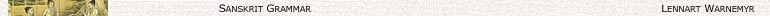Contents

A scheme of vowel gradation is (in principle) acheived as follows:

 VAi = a + GAi ∨ Yia + a (D1) GAi = a + Ai ∨ Yia (D2) Ai = a ∨ ī̆ ∨ ū̆ ∨ ṝ̆ ∨ ḷ (D3)

This can written out (with a few exceptions) as:

 lengthened (vṛddhi) ā ai yā au vā ār rā āl lā full (guṇa) a e ya o va ar ra al la [normal] ā̆ ī̆ ya ū̆ va ṝ̆ ra ḷ la [samprasāraṇa] ī̆ ū̆ ṝ̆ ḷ

Note:

are quoted differently having:

1) A = Ai

2) A = Yia.

The second kind is capable of weakening as seen above. This is called samprasāraṇa.

Notation:

having A in the vṛddhi-row is denoted by V

having A in the guṇa-row is denoted by G

having A in the [normal]-row is denoted by

W denotes:

1) of the first kind in the [normal]-row or

2) of the second kind in the [samprasāraṇa]-row.

WA denotes the A in W.

= 1K A2K which are prosodically long show no gradation